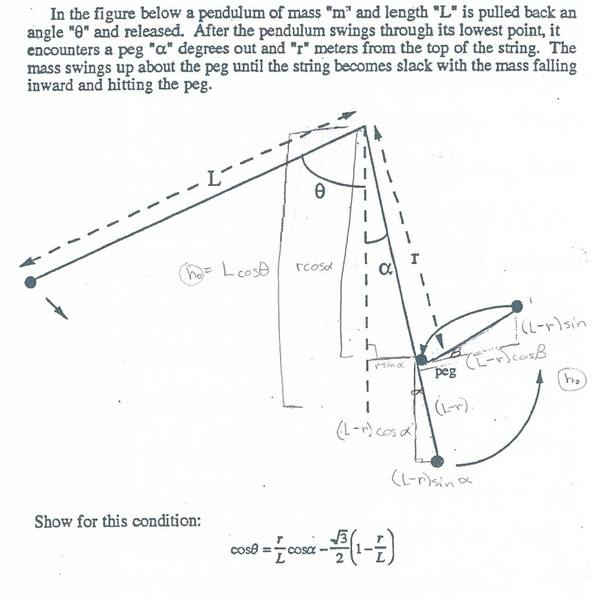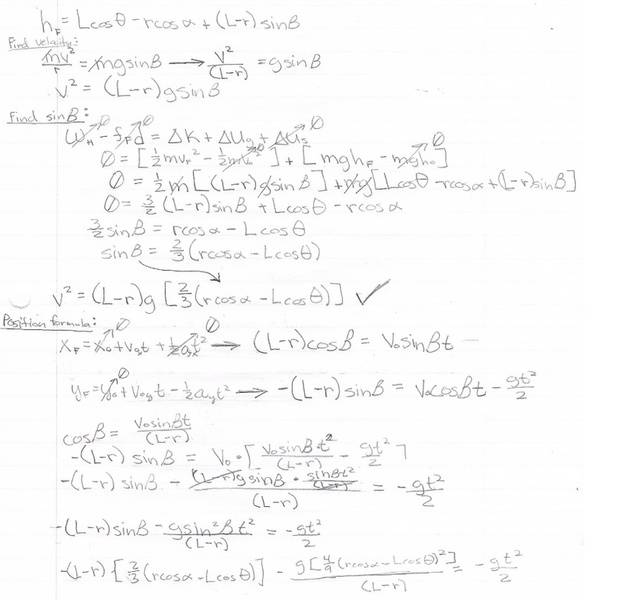# Pendulum swings into a peg

## Homework Statement

With this problem I have to get the answer: cosθ = r/L * cosα - √(3)/2 * (1 - r/L)
which in other words mean I need to find angle θ with arccos[r/L * cosα - √(3)/2 * (1 - r/L)].

Here's the picture:Lcosθ is the vertical length of the string at its lowest point.

rcosα is a fraction of that same vertical string in terms of displacement "r" (which is from the start of the string to the peg)

∠β is the angle between the peg and the horizon.

(L-r) sinβ is the height from the end of the peg and the horizontal

(L-r) cosα is the horizontal length of that same peg.

(L-r)cosα is the vertical length of the string from the ball to the peg.

So, this is not really a physics issue but more like a math issue but since this is a physics problem I've decided to put it under here.

My problem is that I am unable to continue from this point as shown on the picture of my attempt. I don't know where to continue from here on out. I am trying to find "t" for the equation but I am unsure how. Where do I continue from now?

## Homework Equations

[/B]
Newtonian Position Formula:
yf = yi +viyt + .5gt2
xf = xi +vixt + .5gt2

Energy Equation:
Work of hand - force of friction * displacement = delta Kinetic Energy + delta Potential Energy

Wh - fF*d = [.5*mvf2 - .5*mvi2] - [mghf - mghi]

## The Attempt at a Solution

Picture of Attempt:[/B]

BvU
Homework Helper
Hello Stranger,Your position formula is only valid for uniform acceleration. You don't have that here !
Can you think of a condition you can impose on ##\beta## ?

Hello Stranger,Your position formula is only valid for uniform acceleration. You don't have that here !
Can you think of a condition you can impose on ##\beta## ?

Thanks for the welcome :).
The only method I can think of when dealing with changing acceleration by breaking it into parts. Each part for every time the value of acceleration changes. I have not yet learned ho to derive very well but I know it exists. As for angle β I am clueless on what to impose.

BvU